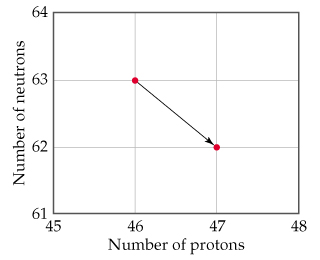# Problem: Write the balanced nuclear equation for the reaction represented by the diagram shown here.

###### FREE Expert SolutionNeutral atom = # protons = atomic number

mass number = # of protons + # of neutrons

Reactant:

# of neutrons = 63
# of protons = 46
mass number = 63 +46 = 109

Element atomic # 46 = Palladium (Pd)

${}_{\mathbf{46}}{}^{\mathbf{109}}\mathbf{Pd}$

Product:

# of neutrons = 62
# of protons = 47
mass number = 62 +47 = 109

Element atomic # 47 = Silver (Ag)

${}_{\mathbf{47}}{}^{\mathbf{109}}\mathbf{Ag}$

84% (292 ratings)###### Problem DetailsWrite the balanced nuclear equation for the reaction represented by the diagram shown here.

Frequently Asked Questions

What scientific concept do you need to know in order to solve this problem?

Our tutors have indicated that to solve this problem you will need to apply the Radioactive Decay concept. You can view video lessons to learn Radioactive Decay . Or if you need more Radioactive Decay practice, you can also practice Radioactive Decay practice problems.

What professor is this problem relevant for?

Based on our data, we think this problem is relevant for Professor Zheng's class at ASU.